# Circuit Parameters and Parameter Sweep for Educators

Publish Date: Jul 17, 2019 | 8 Ratings | 4.38 out of 5 | Print | Submit your review

### 1. Introduction

Circuit parameters provide the ability to define schematic level variables that can then be easily modified to match homework, lab problems and other parametric analysis of a circuit. Circuit Parameters allow us to highlight the key components and variables that characterize the response of a analog and power circuits. Prior to Multisim 13.0 the calculation of important variables and setting of related component values would have been done manually.

With circuit parameters, students can embed variables within a schematic, which can be based on theoretical calculation and cascade to all applicable components. These variables can also be used in expressions so specific circuit characteristics can be determined dynamically based upon other component values within the schematic. This allows students and educators to bridge the gap between theory and the laboratory, as well as opens up to the possibility of far more advanced simulation analysis.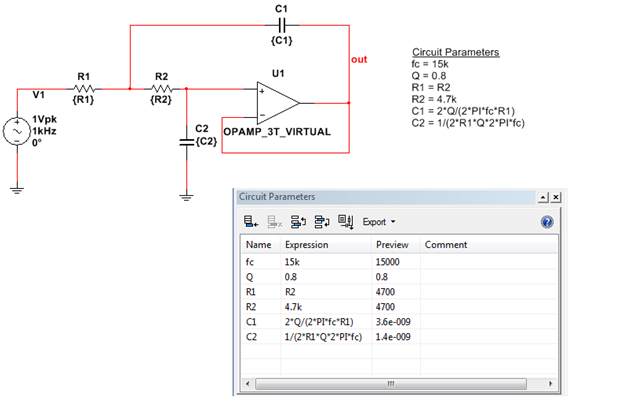The Parameter Sweep has been improved to allow incorporation of circuit parameters as well as enhanced configuration. Students can use the parameter sweep to analyse the circuit with a range of variables to fully understand the affect these have on the circuits’ performance. This tutorial illustrates this through two examples demonstrating how we can use the Circuit Parameters and the improved Parameter sweep to promote the understanding of analog and power circuit behaviour.

### 2. Example 1 – RLC Circuit

To demonstrate the advantages we can gain from using circuit parameters alongside the parameters sweep, we are going to consider a simple RLC circuit as an example. Firstly, we will create the schematic. Once complete, we will then assign circuit parameters to the key variables and components that characterize the circuit. Schematic simulation and the improved parameter sweep analysis model will allow us to gain a comprehensive understanding of the circuit behaviour.

### Step 1 – Build the Simple Analog Schematic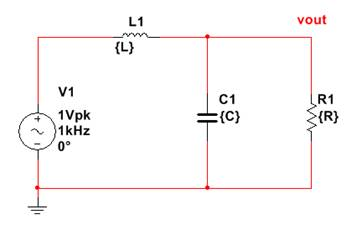1. Place an AC power source on the schematic. Right-click and select Place component from the shortcut menu. Choose the following parameters:

Database: Master Database
Group: Sources
Family: Power Sources
Component: AC_POWER

Select OK to place the component on the schematic. You can modify the peak voltage and the frequency by double-clicking the component. Select a Voltage (pk) at 1 V and a frequency of 1 kHz.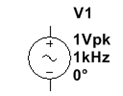2. Place a resistor, capacitor and inductor on the schematic. Leave the default values for these because we will be setting them using Circuit Parameters.

Database: Master Database
Group: Basic
Family: CAPACITOR, INDUCTOR, RESISTOR
Component: C=50 µF, I=20 mH, R=10 Ω

3. Place the circuit ground. In the select a component dialog box, choose the following parameters:

Database: Master Database
Group: Sources
Family: Power Sources
Component: Ground

4. Connect together the components as shown below.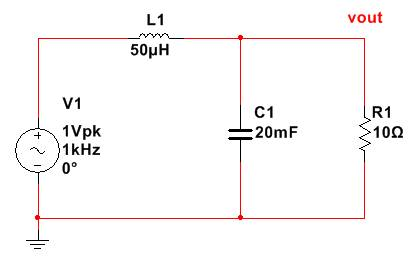5. Define the net that connects the inductor, capacitor and resistor to be vout. Right click the wire connecting these terminals, within the preferred net field name the net: vout. Also select the ‘Show Net Name’ options so this net is labelled on the schematic.

This should complete the schematic and allow us to setup the circuit parameters.

### Step 2 – Assign Circuit Parameters to for Key Variables and Components

In this example we are going to be investigating the effect of two important filter design variables:

• Cut-Off Frequency – frequency at which the amplitude is reduced by a 3dB corner.
• Quality Factor – Dimensionless parameter to describes the degree of damping.

Here we are going to set the value for the inductor, cut-off frequency and the quality factor and dynamically determine the required resistor and capacitor component values.

1. Open the circuit parameters panel through View » Circuit Parameters. This gives us a table through which we can configure our schematic level parameters.

2. Define the following parameters within the table:

 Parameter Name Expression Comment Fc 10k Cut-off Frequency L 10u Inductor Value Q 0.8 Quality Factor

These parameters are going to remain static. The resistor and the capacitor values are going to be determined dynamically based upon these values.

3. In order to determine the resistor and capacitor values we need to enter the corresponding expressions based on analog electronics fundamentals. Enter the following additional circuit parameter values:

 Parameter Name Expression Comment C 1/(2*PI*Fc)^2/L Capacitor Value R Q/sqrt(C/L) Resistor Value

Upon completion of the above expressions you will notice that Multisim calculates a preview of the values that will be used for R and C as in the image below: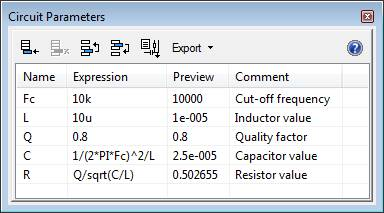4. Whilst we have defined our circuit parameters we still need to assign these to our components within the schematic. To do this, double click on the resistor in the schematic and set its value to be the corresponding circuit parameter name (b in this case) as in the image below. Repeat the process for the inductor and capacitor (with the corresponding L and C circuit parameters).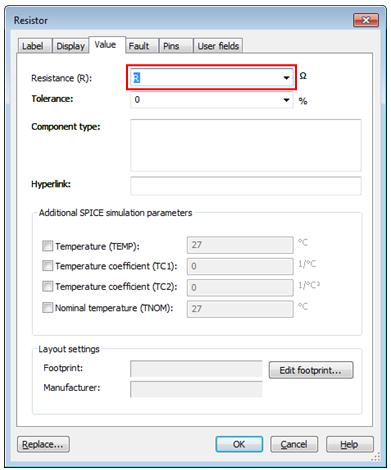Once assigned Multisim will use the circuit parameter value for the assigned components when simulating or running analysis models on the schematic.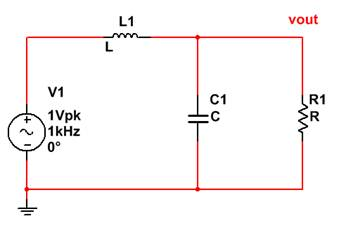5. One very useful aspect of circuit parameters is we can change the value of one component and immediately see the affect this will have on other components. Try changing the value of the cut-off frequency (Fc) from 15k to 10k and view the effect this will have on the preview of the resistor and capacitor values (R and C). This allows students to see the immediate effect of requiring a different cut-off frequency on the capacitor and resistor values. Return the Fc value to 15k.

### Step 3 – Analysis the Schematic to understand the Parameters Effect

With the circuit created we can run analysis models ensure it responds as we expected.

1. Firstly we will run an AC analysis to check the cut off frequency is that which we specified. Select Simulate » Analyses » AC Analysis

2. Keep the defaults for the Frequency Parameters.

3. Click on the Output tab and select V(vout) from the Variables in circuit pane. Click add to use this for the analysis as shown below.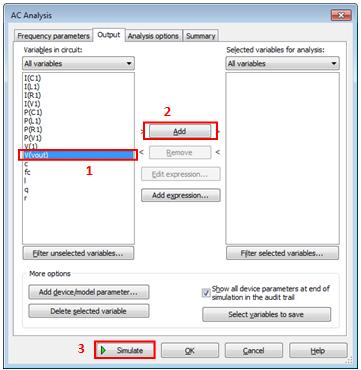4. Click Simulate to start the simulation.

5. This will produce a magnitude and phase graph as below, from which we can see the cut-off frequency is at 15kHz.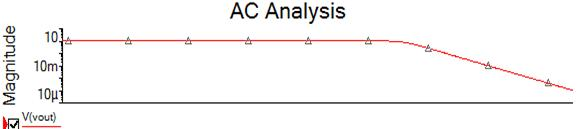6. We can also use circuit parameters to explore how key variables affect the response of a circuit. In this case we will explore the affect the quality factor Q has on the response. We can do this using the Parameter Sweep analysis.

7. Open the Parameter Sweep Analysis dialog box: Simulate» Analyses » Parameter Sweep.

8 .Configure the parameter sweep as displayed in the figure below. This is setup to sweep through a range of values to identify the affect the quality factor has on the AC response of the circuit.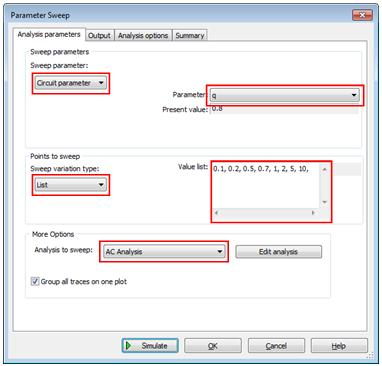9. Click on the output tab and select V(vout) to be the parameter for analysis as you did for the AC analysis previously. Click simulate to run the analysis.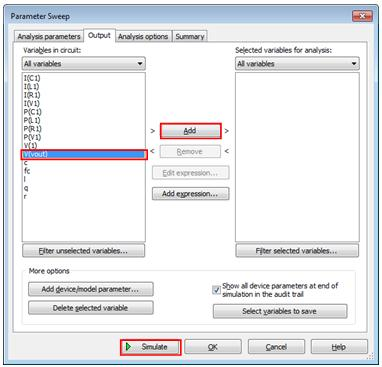10. The results are displayed on a graph allowing us to clearly see the response for various Quality Factor values.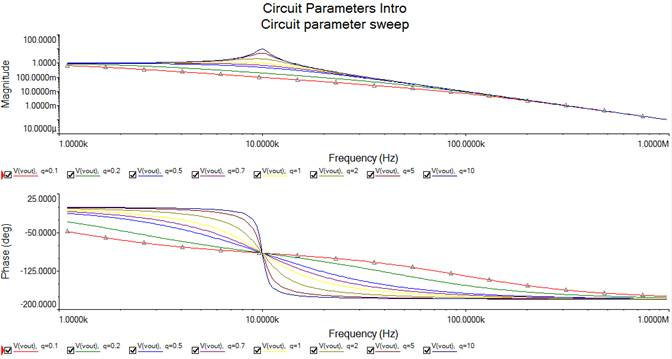### 3. Example 2 – Bipolar BJT Circuit

This example uses circuit parameters to demonstrate the impact that the emitter degeneration resistance can have on the output signal of a BJT Circuit. We will use a parameter sweep to identify the affect different resistances will have.

1. Copy the snippet below into Multisim which is an example of a simple BJT circuit.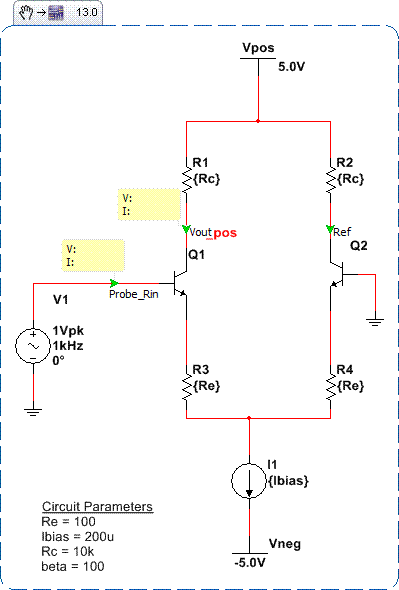2. If not already open, view the circuit parameters window vial View » Circuit Parameters.

3. Define the circuit parameters as shown in the window below. These have already been assigned to the components in the schematic so be sure to use the identical names as in the image below. In doing this we are setting the two emitter resistors and collector resistors using a single circuit parameter. This allows us to perform a parameter sweep that will cascade to both resistors.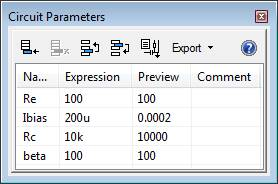4. Run a Parameter Sweep: Simulate » Analysis » Parameter Sweep. Configure the dialog box as below. This will perform a Single Frequency AC Analysis Sweep which is one of the enhancements in the Parameter Sweep Analysis. We will sweep through various values of Re at a single frequency.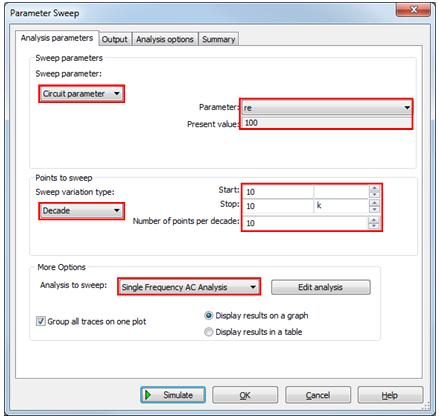5. Click the Edit Analysis button. Change the output to display the Magnitude / Phase as shown in the image below. Click OK.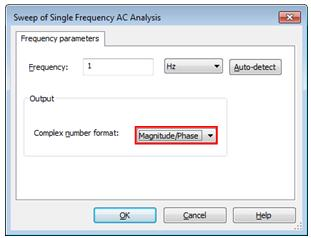6. Switch to the Output tab. Here we want to set the output as the gain (Vout1 / Vref) allowing us to view the gain at different emitter resistance values. On the Output tab click Add Expression.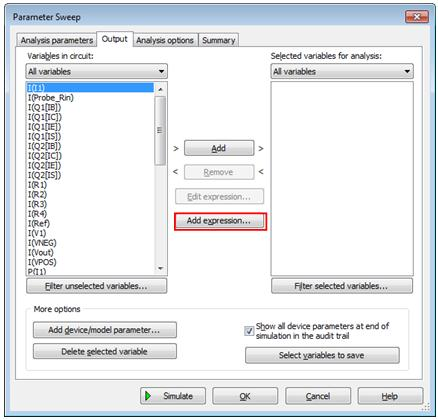7. Enter the expression for the gain of our schematic: V(Vout)/V(Ref) using the variables and the functions. Select OK once the expression is complete.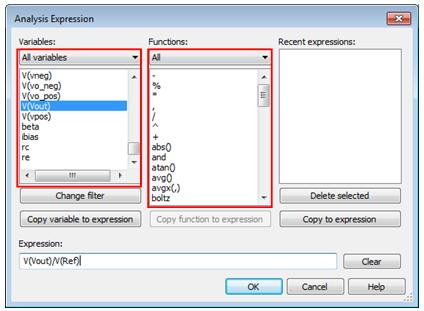8. Returning to the Output tab ensure that the gain expression is selected as a variable for analysis. Click Simulate.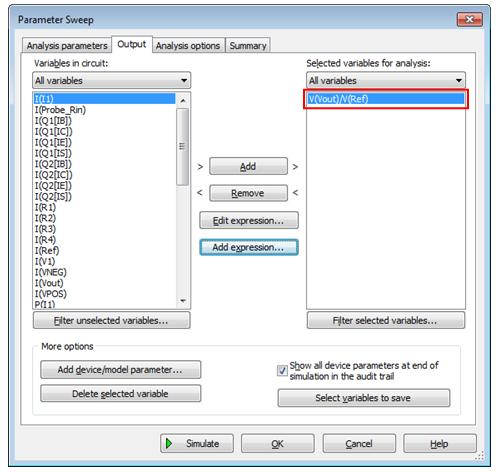9. The resultant graph will illustrate that as we increase the resistance at the emitter we achieve an increase in the gain. This can be demonstrated because using circuit parameters we can change the value of multiple components simultaneously.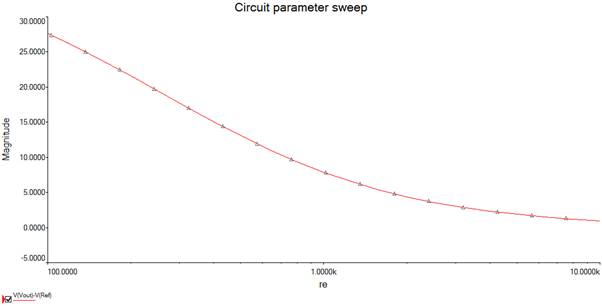10. To fully understand this problem we also need to look at the affect this has on the input resistance: R(Probe_Rin) / I(ProbeRin).

11. Return to the configuration window for the Parameter Sweep analysis and select the Output tab. As before click Add Expression and enter the expression V(Probe_Rin) / I(ProbeRin) as below.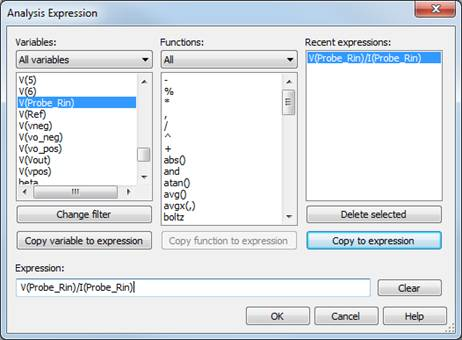12. Run the analysis and we can identify that with an increase in resistance and the emitter we have the effect of and increase resistance at the input of the amplifier.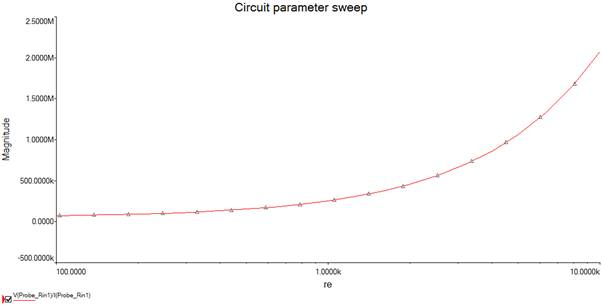This example allows the students to understand that there is a trade of in the design when increasing the emitter resistance.

# Conclusion

Circuit parameters are a key feature for educators in teaching analog and power theory. They provide schematic level variables that the user can assign to components, calculate key variables and use in analysis models. Together these allow students to gain a deeper understand and experiment with schematics to understand the full characterization of the circuit.

### Ratings

Rate this document